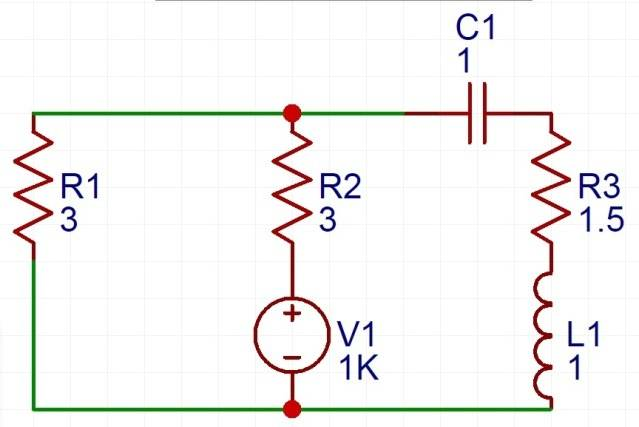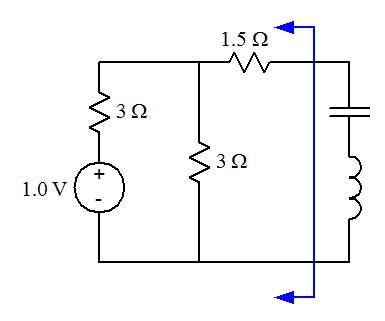# How do I find the Thevenin Equivalent of this circuit?

• Engineering
• D4rk

## Homework StatementI need to find the Thevenin Resistance and the Thevenin Voltage

## Homework Equations

Vth =Rth I (Short Circuit)

## The Attempt at a Solution

I know the answer is supposed to be Rth = 3Ω and Vth = 0.5V, but I couldn't get there with my method.
I only get the Rth to be 3 Ω if I first find the parallel equivalent of the two 3 Ω resistors and then have that be in series with the 1.5Ω resistor. If I try it the other way around though ( have the 1.5Ω resistor be in parallel with the 3Ω resistor, I get a different answer of 4 Ω.

Also I don't really understand how the Thevenin voltage would be found in this case as observed by the source because won't the absence of the voltage source cause the Vth to be 0V?

Help on this matter would be very much appreciated.

Since you have not indicated the 2 nodes at which the Thevenin equivalent is to be taken, this is an incomplete problem statement and thus has no solution.

Is that voltage source 1V or 1000V?

Perhaps L represents the load, rather than an inductance?

Since you have not indicated the 2 nodes at which the Thevenin equivalent is to be taken, this is an incomplete problem statement and thus has no solution.

That was also one of the problems I was facing, the problem itself didn't include the nodes at which the Vth is to be taken. I was going under the assumption that it might be at the voltage source. Is that possible? Can there be a Vth without a voltage source?

Is that voltage source 1V or 1000V?

Perhaps L represents the load, rather than an inductance?
Sorry about that, I rebuilt the circuit in a simulator and didn't see that 1K looming by the source. The voltage source is 1V.

I rebuilt the circuit in a circuit simulator, the problem stated that L1 was an Inductor. Rather L1 was a series of inductors that was supposed to be simplified.

You would get your answer if L were to be regarded as the load, so I suggest you do that.

That was also one of the problems I was facing, the problem itself didn't include the nodes at which the Vth is to be taken. I was going under the assumption that it might be at the voltage source. Is that possible? Can there be a Vth without a voltage source?
It would be unusual for that to be the case. Normally one takes the Th Eq of a circuit that contain either a current source or a voltage source. The fact that you are asked for the Th Voltage pretty much rules it out as a normal problem statement, while not totally ruling it out technically.

It would be unusual for that to be the case. Normally one takes the Th Eq of a circuit that contain either a current source or a voltage source. The fact that you are asked for the Th Voltage pretty much rules it out as a normal problem statement, while not totally ruling it out technically.
Might be that I'm interpreting it incorrectly. The original question is as follows:
"Determine the Thevenin equivalent of the sources and resistor in the circuit."

Also, another question I was wondering about was with regard to the Thevenin Resistance. Shouldn't the answer in this case be the same regardless of whether we take the combination of the 3Ω resistors to be in series with the 1.5Ω or if we regard the combination of the 3Ω and 1.5Ω to be in parallel with the 2nd 3Ω resistor?

Might be that I'm interpreting it incorrectly. The original question is as follows:
"Determine the Thevenin equivalent of the sources and resistor in the circuit."
Exactly my point. You are asked to find the Th Eq voltage. If the voltage source is considered to be the load, the Th Eq voltage is zero, which would be an unusually simple problem.

Also, another question I was wondering about was with regard to the Thevenin Resistance. Shouldn't the answer in this case be the same regardless of whether we take the combination of the 3Ω resistors to be in series with the 1.5Ω or if we regard the combination of the 3Ω and 1.5Ω to be in parallel with the 2nd 3Ω resistor?
When a circuit is simple enough that there's nothing in it but a loop with two resistors, then it is meaningless whether you call them series or parallel. They are both.

•D4rk
Exactly my point. You are asked to find the Th Eq voltage. If the voltage source is considered to be the load, the Th Eq voltage is zero, which would be an unusually simple problem.

When a circuit is simple enough that there's nothing in it but a loop with two resistors, then it is meaningless whether you call them series or parallel. They are both.
This problem was very poorly asked then. I get the gist of it now though. Thanks for your help :).

Also I drew a few scenarios I had in my head and I better understand what you are saying about the resistors. Once again, thanks for the help :). Much appreciated.

Also, another question I was wondering about was with regard to the Thevenin Resistance. Shouldn't the answer in this case be the same regardless of whether we take the combination of the 3Ω resistors to be in series with the 1.5Ω or if we regard the combination of the 3Ω and 1.5Ω to be in parallel with the 2nd 3Ω resistor?
The 1.5-ohm resistor isn't in parallel with the other resistors, so analyzing the circuit as if it were in parallel with the 3-ohm resistor is pointless and misguided.

You can redraw the circuit, moving some of the components around a bit without changing the behavior of the circuit. In particular, isolate the resistor network from the reactive load components and make the voltage divider nature of the resistor network more obvious:Take the Thevenin equivalent where the blue arrow divides the source and resistor network from the reactive load components.